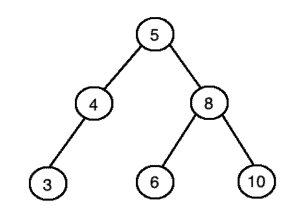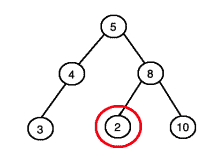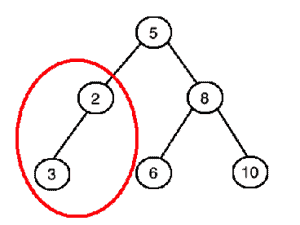## 1. Introduction

In this article, we’ll discuss the problem of validating a binary search tree. After explaining what the problem is, we’ll see a few algorithms for solving it. Then we’ll see the pseudocode for these algorithms as well as a brief complexity analysis.

## 2. Problem Explanation

We’re given as input a binary tree and would like to determine whether it’s a valid binary search tree. In other words, we’ll need to check four things:

• Is every node value in the root’s left subtree less than the root’s value?
• Is every node value in the root’s right subtree greater than the root’s value?
• Is the root’s left subtree also a binary search tree?
• Is the root’s right subtree also of a binary search tree?

If these four conditions are met, then we can be sure that the binary tree is a binary search tree. For example, this tree is a binary search tree since the conditions are met:Whereas this tree is not a binary search tree since we have a node value (circled in red) in the right subtree of the root which is less than the root’s value:Finally, this tree is not a binary search tree because the left subtree of the root (circled in red) is not a binary search tree:## 3. Algorithms

### 3.1. Naive Approach

An extremely naive approach would be to traverse the tree and check at each node whether the left child’s value is smaller and the right child’s value is bigger. This approach is wrong because it’ll only work on certain trees, not all trees.

A correct naive method (which is suggested by the conditions in the previous section) is this:

• If the tree is a single node, return true
• Traverse every node in the left subtree, checking whether each value is smaller than the root’s value
• Traverse every node in the right subtree, checking whether each value is larger than the root’s value
• If we found a node in the left subtree whose value is bigger than the root’s or a node in the right subtree whose value is smaller than the root’s, then return false
• Recursively check whether both the left and right subtrees of the root are also binary search trees and if yes then return true

### 3.2. Efficient Method Using Upper and Lower Limits

The previous algorithm is too slow and we can do better. One possibility is to keep track of upper and lower limits for each node as we traverse the tree. At each node, we’ll check whether that node’s value falls within the current limits.

Whenever we recurse on a left subtree we’ll use the current node’s value as the new upper limit and keep the original lower limit. If we recurse on a right subtree, we’ll use the current node’s value as the lower limit and keep the original upper limit. The inputs to the algorithm are(we’ll begin with the root),, and.

So, let’s outline this in detail:

• Ifequals, return• Ifdoes not equaland the value atis less than or equal to, return• Ifdoes not equaland the value atis greater than or equal to, return• Recursively check that the left subtree ofis a binary search tree, with the value ofas the upper limit andas the lower limit. If the left subtree is not a binary search tree then return.
• Recursively check that the right subtree ofis a binary search tree, with the value ofas the lower limit andas the upper limit. If the right subtree is not a binary search tree, then return.
• ReturnThe above algorithm is much faster than the previous one because we only visit each node exactly once.

### 3.3. Efficient Method Using Inorder Traversal

There is another algorithm we can use to solve this problem. We can do an inorder traversal of the given tree, storing the node values in a list, and then checking whether the list is sorted or not.

If an inorder traversal produces a sorted order, then the tree must be a binary search tree. But why is this the case and how can we know for sure? We can answer these questions using mathematical induction.

The base case is that if we have a single node then the statement holds. Inorder traversal of a single node will always return a sorted order, and a single node will always be a binary search tree.

Now we can inductively assume that the statement holds for any left subtree and any right subtree. Using this inductive hypothesis along with the base case, we can show that the original statement holds.

If the inorder traversal of our tree returns a sorted order, then the inorder traversal of the left and right subtrees must’ve been in sorted order as well. This is because the inorder traversal will always traverse the entire left subtree, then the root, and finally the entire right subtree.

This also means that every node value in the left subtree must be smaller than the root’s value, and every node value in the right subtree must be larger than the root’s value. Also, we know inductively that both the left and right subtrees must be binary search trees, so we’re done with our proof.

This inorder traversal based method can be further improved by noticing that we do not have to store all the node values in a list. We can simply always check whether the previous element in the traversal is less than the current element.

## 4. Pseudocode

Here we’ll show pseudocode for four different algorithms.

### 4.1. Naive Algorithm

The algorithm below is the naive approach where we first traverse the left and right subtrees and then recursively check whether the two subtrees are binary search trees:In the above code, we can see that there are two functions:and.takes as input the root node of a binary tree and returnsif the given binary tree is a valid binary search tree. In line 3, we have a condition that checks whether all the node values in the left subtree are smaller than the root’s value and all the node values in the right subtree are larger than the root’s value.

If this condition is met then on line 4 we recursively check whether both the left and right subtrees are also binary search trees. Otherwise, we return. Finally, we returnif the root is equal to.

The functiontakes as input the root node of a subtree, the value that we want to compare all the subtree values to, and a boolean variable which tells us whether the values in this subtree should be less than or greater the given value. This function iterates through every node of the given subtree and checks whether all the nodes in the subtree are less than or greater than the given value (depending on whetherisor).

### 4.2. Efficient Algorithm Using Upper and Lower Limits

Here, we’re using the idea of lower and upper limits and traverse each node exactly once:Algorithm 2 first checks whether the tree is empty. If it is we returnsince an empty tree is a valid binary search tree.

Then in lines 5 through 10 we have twostatements that check whether the current node’s value is within the lower and upper bounds.

Lines 11 through 16 contain twostatements which recursively check whether the left and right subtrees also obey the lower and upper bounds. Note that for the left subtree the new upper bound is the current node’s value, and for the right subtree the new lower bound is the current node’s value.

Finally, we returnif we get past all thestatements since this means the tree must be a binary search tree.

### 4.3. Efficient Algorithm Using Inorder Traversal

This algorithm uses an inorder traversal and stores all the node values in a list. Then it checks whether the list is sorted:### 4.4. Space-Optimized Inorder Traversal Based Algorithm

The algorithm below is an optimized version of the above algorithm in the sense that it does not use an extra list to store the values:In this last algorithm, the functiontakes as input the root node, an arraywhich stores the previous node in the traversal as its only element, and an arrayof one boolean which indicates whether the tree is valid or not.

Initially, the boolean variable insideis set to true. If at any point in the traversal the previous node’s value is greater than or equal to the current value, then the boolean inis set to false and we quit thefunction by returning.

## 5. Complexity

The naive algorithm is the slowest of all four algorithms. Its time complexity is equal towhere n is the number of nodes in the tree.

We’ll show that the naive algorithm has a worst-case time complexity ofby showing an example where it isand then showing that it cannot be worse than.

What is a case when the running time is? One possibility is if the tree happens to be a path of length. Since we’re iterating through all the descendants of each node, the total number of times we visit some node in such a path is equal to. This is because in a path of lengththe root node hasdescendants, the second node hasdescendants, and so on.

How do we know that the worst-case complexity of the naive algorithm cannot be worse than?

The maximum number of descendants possible for any node issince there arenodes in the tree. So even if every node haddescendants, we would still only haveiterations in the algorithm. This is still.

The algorithm which uses upper and lower limits has a time complexity ofsince we visit each node exactly once.

In the inorder traversal based algorithm, we perform an inorder traversal which takestime. Then we check whether a list is sorted which also takestime. This gives us an overall time complexity of.

Finally, the space-optimized inorder traversal based algorithm has a time complexity ofsince we’re simply doing an inorder traversal with constant-time operations.

## 6. Conclusion

In this article, we have seen four different algorithms for validating a binary search tree. We have also seen pseudocode for these algorithms as well as a time complexity analysis. Our optimal solution for this problem runs intime.